A multiple-relaxation-time lattice Boltzmann method for high-speed compressible flows*
National Key Laboratory of Science and Technology on Aerodynamic Design and Research, Northwestern Polytechnical University, Xi’an 710072, China

Corresponding author. E-mail: zhongcw@nwpu.edu.cn

*Project supported by the Innovation Fund for Aerospace Science and Technology of China (Grant No. 2009200066) and the Aeronautical Science Fund of China (Grant No. 20111453012).

Abstract

This paper presents a coupling compressible model of the lattice Boltzmann method. In this model, the multiple-relaxation-time lattice Boltzmann scheme is used for the evolution of density distribution functions, whereas the modified single-relaxation-time (SRT) lattice Boltzmann scheme is applied for the evolution of potential energy distribution functions. The governing equations are discretized with the third-order Monotone Upwind Schemes for scalar conservation laws finite volume scheme. The choice of relaxation coefficients is discussed simply. Through the numerical simulations, it is found that compressible flows with strong shocks can be well simulated by present model. The numerical results agree well with the reference results and are better than that of the SRT version.

Keyword: 05.20.Dd; 47.11.Df; 47.40.−x; lattice Boltzmann method; multi-relaxation-time; compressible flow; finite volume method
1. Introduction

The lattice Boltzmann method (LBM) is a modern approach in computational fluid dynamics. Unlike the traditional method that was based on macroscopic equations, the LBM is based on solving the discrete-velocity Boltzmann equation and macroscopic quantities are obtained by the statistics of distribution functions. The appealing merits of the LBM are a simple algebraic operation, linear convective terms, and easy parallelisation. Because of these advantages, LBM has been successfully applied in many areas of fluid mechanics, such as in microflows,  turbulent flows,  multiphase flows,  blood flows,  heat transfer flow,  etc.

Continuous efforts have been made to improve the LBM since its inception. Besides the single-relaxation-time (SRT) scheme, which has been routinely used, there are several variations of LBM, including the entropic scheme, [20, 21] the two-relaxation-time (TRT) scheme, [22, 23] and the multiple-relaxation-time (MRT) scheme.[24, 25] It should be mentioned that the MRT scheme has attracted much attention in recent years. It is more suitable than SRT scheme in terms of physical principles, parameter selection, and numerical stability. Moreover, when the transformation matrix and moments of MRT scheme are defined, the formulations of equilibrium distribution functions are fixed.

Generally, the LBM model with two-dimension-nine-velocity (D2Q9) lattice and advection-collision evolution process is called the standard LBM. The standard LBM model can only recover the incompressible Navier– Stokes equations. In 1993, Alexander et al. and Qian proposed the multispeed (MS) approach which is a straightforward extension of standard LBM. The models proposed in Refs.  and  have several drawbacks, such as nonlinear error terms from macroscopic equations, fixed Prandtl number and specific-heat ratio, and low Mach number limit. Chen et al. eliminated the nonlinear error terms by considering higher-order constraints. To obtain adjustable specific-heat ratio, Yan et al. proposed a multi-speed and multi-energy-level model. Sun,  Shi et al.,  Kataoka and Tsutahara, [34, 35] and Watari devised several models that introduced an extra energy into the definition of total internal energy. In 1998, He et al. proposed the first double-distribution-function (DDF) model. In this model, the DDF is used for the flow field and additional internal energy distribution function is used for the temperature field. The DDF model has adjustability for the Prandtl number and specific-heat ratio. Guo et al. proposed a total energy distribution function to replace the internal energy distribution function. Li et al. introduced circular function into the DDF model and achieved satisfactory simulation results in high Mach number flows. On the basis of previous researches, we proposed a potential energy DDF model which keeps the part of the MS approach. During this period, the finite difference and finite volume method are widely used in LBM to obtain more stable and accurate results.

Because of the advantages compared to the SRT model, MRT LBM models have been successfully applied to compressible flows. Zheng et al. developed an MRT LBM which has an adjustable Prandtl number by modifying the collision term of some moments. Chen et al. transferred the model of Kataoka and Tsutahara from SRT LBM to MRT LBM. In this paper we improved the potential energy DDF model by introducing MRT scheme into the evolution of density distribution functions. The moment space and corresponding transformation matrix are constructed for the D2Q17 lattice. Equilibria of the nonconserved moments are chosen so as to recover the complete compressible Navier– Stokes equations through the Chapman– Enskog analysis. Finally, the capability of this model is compared with previous SRT model through several compressible cases that test its robustness.

The rest of the paper is organized as follows. The compressible model and numerical method are described in Section 2 and Section 3, respectively. Section 4 presents selected numerical simulations including the Colella explosion wave and flows round a circular cylinder. The obtained results are compared with available computational results. The last section is dedicated to the concluding remarks.

2. LBM model
2.1. DDF LBM model

The discrete Boltzmann Bhatnagar– Gross– Krook (BGK) equation is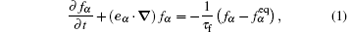where α = 0, 1, 2, … , N is the discrete velocity direction, fα is the density distribution function,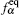is the corresponding equilibrium distribution function, N is the total number of discrete velocity directions, eα is the discrete velocity, τ f = μ / p is the relaxation time, and p = ρ RT is the pressure.

To have an adjustable specific-heat ratio and Prandtl number and recover the complete Navier– Stokes equations, the potential energy distribution functions are introduced and the corresponding governing equation is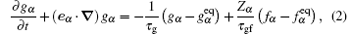where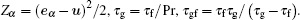The macroscopic quantities (density, momentums, and total energy) are calculated by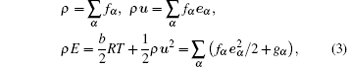where b is the number of degrees of freedom (DOF).

The potential energy equilibrium distribution function can be simply obtained by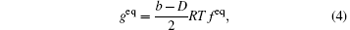where D is the number of dimensions.

In Ref.  the D2Q17 (see Fig. 1) circle function which is proposed by Qu et al. is used. The circular function assumed that all mass, momentum, and energy are concentrated on a circle. The circular function is then distributed amongst the lattice velocities through Lagrangian interpolation with a set of base vectors related to the discrete velocities. The discrete velocities are defined as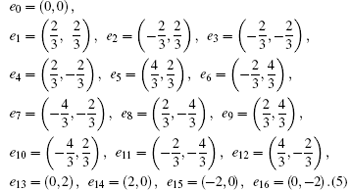Figure Option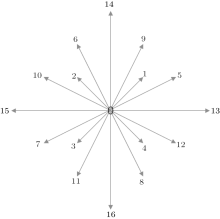Fig. 1. D2Q17 discrete velocity model.

2.2. Corresponding MRT model

For convenience, the density distribution function space is denoted by f in the following. To construct the MRT model, a moment space m, a N × N transformation matrix M, and a diagonal relaxation matrix S = diag(s0, s1, … , sN) to replace the relaxation coefficient in Eq. (1) are defined. The relationship between the distribution function space and the moment space is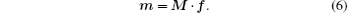For the D2Q17 model, m is defined specifically as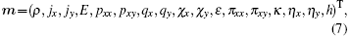where density ρ and the momentum (jx, jy) are conserved moments. Other moments are non-conserved moments and their equilibria are functions of the conserved moments in the system. Among them, E is related to the kinetic energy, qx and qy are proportional to the x and y components of the energy flux, and pxx and pxy are proportional to the diagonal and off-diagonal components of the viscous stress tensor.[47, 48]

The matrix M is composed by a set of vectors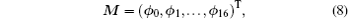where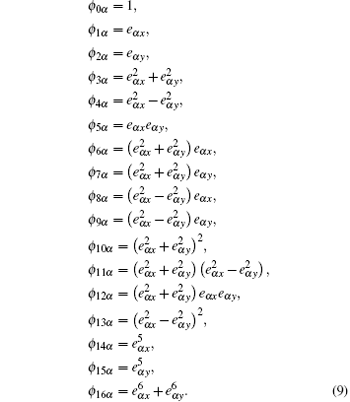We find that the same density distribution functions in Refs.  and  can be obtained when the corresponding equilibria of the moments are defined as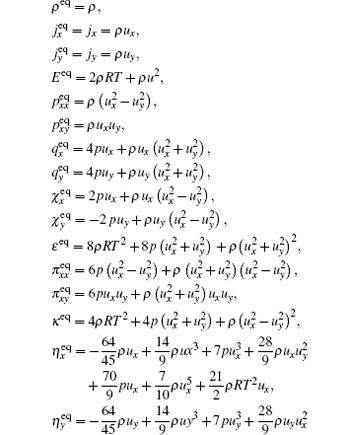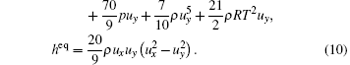Finally, the governing equations become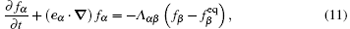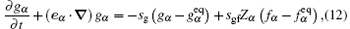where Λ = M− 1SM is the collision matrix.

Through the Chapman– Enskog analysis, the complete compressible Navier– Stokes equations can be recovered when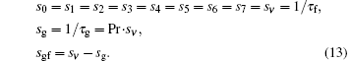There are two points about the governing equations to be concerned. The first one is that MRT model is not used in Eq. (12). The reason is that it is an additional equation and its only role is to implement the evolution of the potential energy distribution functions. Actually, the result will get worse if the MRT model is used. The second point is that no moment appears in Eq. (11). The moments are just intermediate variables used in the modeling process. This is of benefit because it saves memory during computing.

3. Numerical methods

In standard LBM, the discrete velocities are coupled with configuration space and the evolution is carried out in a fixed way. Hence, standard LBM is hard to be applied on a non-uniform grid. To overcome this problem, many people chose to use finite difference or finite volume methods to solve the discrete Boltzmann BGK equation. Although the increase of complexity goes against the computational efficiency, finite difference or finite volume method makes LBM suitable for non-uniform grid and are also more flexible. There are a wide assortment of numerical schemes to choose from.

In this study, the third-order monotone upwind schemes for scalar conservation laws (MUSCL) finite volume scheme were used.[53, 54] Take the kinetic equation of density distribution function for example, equation (11) can be rewritten as[1, 52]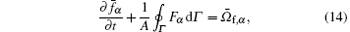where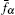is the average distribution function on a cell, A is the area of the cell, Γ is the corresponding cell-boundary,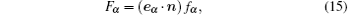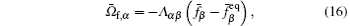and n is the outward-directed unit normal vector.

For a two-dimensional structured mesh, the semi-discretized form of Eq. (14) is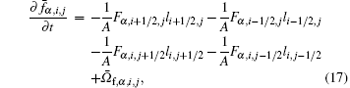where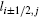and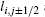are the length of corresponding interface (see Fig. 2),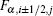and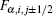are numerical fluxes on the interfaces of a cell (i, j).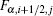is defined as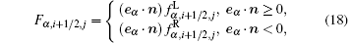where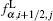and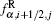are the distribution functions just on the left and right sides of the interface (i + 1/ 2, j).andare determined by the third-order MUSCL with the smooth limiter to extrapolate the value of fα on the two sides of an interface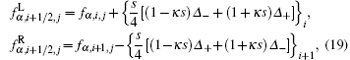where κ = 1/ 3 and s is the van Albada limiter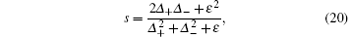where ε is a small number to prevent the division by zero error in the region of zero gradient, and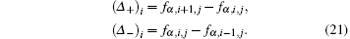For simplicity, the Euler forward scheme is applied for the temporal discretization. The solution for g follows the same method as f.

 Figure Option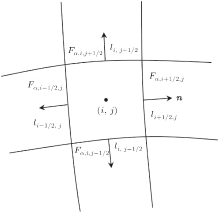Fig. 2. Numerical flux on the interfaces of a cell.

4. Numerical simulations

In this section, we conduct a series of numerical simulations to validate the compressible MRT LBM model. The testing problems include Colella explosion wave and flows around a circular cylinder. In present simulations, the characteristic temperature Tc is often set to a value which is a little bit larger than the maximum stagnation temperature in the whole flow field. Throughout this section, the reference density, velocity, temperature, and length are all taken as 1.0.

4.1. Colella explosion wave

The Colella explosion wave is a strong temperature discontinuity problem that can be used to study the robustness and precision of numerical methods. As a one-dimensional problem, the size of the grid is Nx × Ny = 401 × 6. The top and bottom boundaries are periodic, and the inlet and outlet are set to the equilibrium. In this part, the specific-heat ratio is γ = 1.4, the Prandtl number is 0.71, and the viscosity is μ = 2 × 10− 5.

The initial condition of the Colella explosion wave is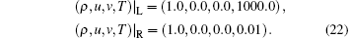Subscripts “ L” and “ R” represent macroscopic variables on the left and right sides of the discontinuity. The time step is Δ t = 1 × 10− 5 and the characteristic temperature is Tc = 1000.

There are nine adjustable relaxation coefficients in this MRT model. According to the physical background described in Section 2.2, these adjustable relaxation coefficients can be taken as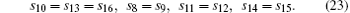In order to measure the error between simulated and analytic results, the normalized mean square errors (NMSE) of density is used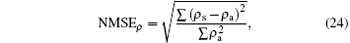where ρ s is the simulated result, and ρ a is the analytic solution. For the SRT case, NMSEρ = 0.2284.

Table 1 shows the values of NMSEρ for some specific values of adjustable relaxation coefficients. It can be seen that the range of the value of adjustable relaxation coefficients which makes the program convergent from 102 to 105 order of magnitude. In this range, NMSEρ first decreases and then increases for all four types of the relaxation coefficients in Eq. (23), and the decrease is faster than the increase. The minimum values of NMSEρ are 0.1782, 0.2035, 0.1775, and 0.2710 for s10, 13, 16, s8, 9, s11, 12, and s14, 15, respectively. The first three values are less than the value of SRT case, but the last one is greater. The corresponding density curves compared with the analytic solution are given in Fig. 3. The fourth curve corresponding to s14, 15 is obviously the worst one. Both the second and third curves have oscillation in the expansion wave and the third curve even has a sharp peak on the left of the shock. Finally, the first one becomes the best choice. So we set s10, 13, 16 to 6000 and the rest relaxation coefficients are equal to sν in the following. In addition, we also find that the optimal value of s10, 13, 16 stays at 6000 when sν changes. This means that the variation of s10, 13, 16 is a general law and can be used in other situations.Table 1. The normalized mean square errors (NMSE) of the density. The relaxation coefficients which are not mentioned are equal to sν in each case; × indicates failed calculation.

 Figure Option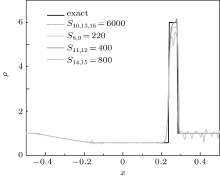Fig. 3. Density profiles of Colella explosion wave for different relaxation coefficients at t = 0.012.

Figure 4 shows the computed pressure, density, temperature, and velocity profiles at t = 0.012, where the SRT results, the MRT results, and the analytical solutions are shown together for comparison purpose. Both the SRT and MRT results capture the shock wave and contact discontinuities well. Because the increasing discontinuity of the initial data immediately spreads out, there is an oscillation of pressure, temperature, and velocity at the right of rarefaction wave. From the density profiles, it can be seen that the MRT results are obviously better than the SRT results. For the pressure, velocity, and temperature, there are no obvious differences.

It is noted that the adjustable relaxation coefficients are analyzed separately. A better result may be found by considering the interaction of relaxation coefficients. However, this is a complex work. The present results have already made a significant improvement.

 Figure Option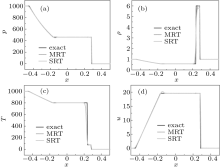Fig. 4. (a) Pressure, (b) density, (c) temperature, and (d) velocity profiles of Colella explosion wave at t = 0.012.

4.2. Flow around a circular cylinder

In this work, the flows around a circular cylinder are presented at two states. The Mach numbers are 1.7 and 5.0, respectively. The Reynolds number is 2 × 105. The specific-heat ratio is 1.4, and the Prandtl number is 0.71. The H-type grid (see Fig. 5) used in both cases is generated by using the following equation: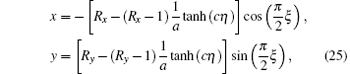where Rx and Ry are the x-direction and y-direction radius of computational domain, respectively, a = tanh (c) with c = 2.5 being a parameter controlling the distribution of the grid. ξ and η are the coordinates of calculation plane.

The left inlet is set to the equilibria of the initial condition. At the right outlet, the first-order extrapolation is used for unknown distribution functions. A symmetric boundary condition is applied at the bottom boundary. For the non-slip boundary condition at the surface of the cylinder, the non-equilibrium extrapolation scheme is used. This method is of second-order accuracy. As shown in Fig. 6, xb is a boundary node, and xf is the closest node of xb in fluid field. Then, the distribution function at xb is set to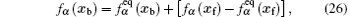where the second part in the bracket on the right-hand side is the non-equilibrium part of the distribution function at xf, which is used to approximate the value at node xb.

 Figure Option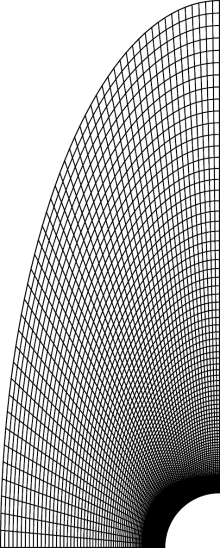Fig. 5. Grid for supersonic flow past a circular cylinder.

 Figure Option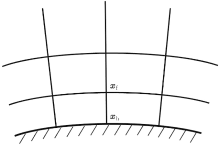Fig. 6. The diagram of wall boundary. xb is a wall node and xf is the fluid node next to the wall.

As mentioned before, the variation of adjustable relaxation coefficients is unaffected by the viscosity. Hence, s10, 13, 16 is also set to 6000. Meanwhile, the other relaxation coefficients are equal to sν .

The first case was simulated in Refs.  and  and can be a reference example to verify the accuracy of the code. In this case, Rx = 4 and Ry = 10, the grid size is 101 × 101, the time step is Δ t = 1 × 10− 5. Figure 7 shows pressure contours in the flow field. We can see that a bow shock is formed in the upstream of the cylinder. The position of the shock is about x = − 3, which agrees with that in Ref. . The reason for the numerical oscillation in the front of the shock is that the grid resolution near the left boundary is coarse.

For a more careful examination, figure 8 depicts the pressure coefficient distribution on the surface of the circular cylinder. The pressure coefficients obtained in this study are in a very good agreement with the numerical reference results. The present results are also higher than the experimental results.

The second case is a high-speed compressible flow. It is predicted that the shock is closer to the circular cylinder. Hence, the computational domain is smaller than that in the first case, Rx = 2 and Ry = 4. The grid size is 151 × 151, and the time step is Δ t = 1 × 10− 6. The pressure contours are shown in Fig. 9. As expected, there is a shock wave at − 1.5 of the x axis. It can be seen that the numerical oscillation in the front of the shock is stronger than preceding case and the

 Figure OptionFig. 7. Pressure contours in the vicinity of the circular cylinder at Ma = 1.7.

 Figure OptionFig. 8. Distribution of surface pressure coefficients around the circular cylinder at Ma = 1.7.

contours have a little deformation near the shock. This means that the present case is close to the limit of the proposed MRT model. The simulation fails when the SRT model is used. It also proves that the MRT model is better than the SRT model.

Figure 10 shows the pressure ratio between the local pressure and the static pressure p/ pstag along the circular cylinder surface. The results are in a good agreement with the results from Refs.  and .

 Figure Option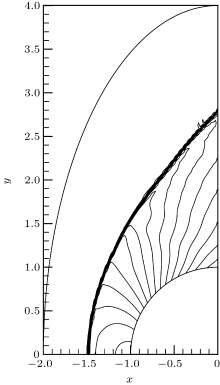Fig. 9. Pressure contours in the vicinity of the circular cylinder at Ma = 5.0.

 Figure Option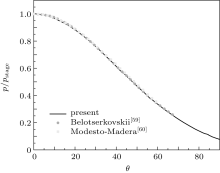Fig. 10. Surface pressure distribution around the circular cylinder at Ma = 5.0.

5. Conclusion

In this paper, we transpose the potential energy DDF LBM to an MRT model. This model can also recover the complete compressible Navier– Stokes equations with a flexible specific-heat ratio and Prandtl number. With the determination of the equilibria of moments, the same distribution functions with the circle distribution functions are obtained. With the finite volume scheme, numerical experiments include Colella explosion wave and two cases of flow around a circular cylinder are simulated. All of the numerical results obtained from this present approach satisfactorily agree with the analytical solutions or simulation data reported in the available literature. Compared with the SRT model, the present MRT model is more accurate and stable.

Reference
 1 Succi S 2001 The Lattice Boltzmann Equation for Fluid Dynamics and Beyond 1st edn. New York Oxford University Press [Cited within:2] 2 Lim C Y, Shu C, Niu X D, Chew Y T 2002 Phys. Fluids 14 2299 DOI:10.1063/1.1483841 [Cited within:1] 3 Toschi F and Succi S 2005 Europhys. Lett. 69 549 DOI:10.1209/epl/i2004-10393-0 [Cited within:1] 4 Zhuo C and Zhong C 2013 Phys. Rev. E 88 053311 DOI:10.1103/PhysRevE.88.053311 [Cited within:1] [JCR: 2.313] 5 Liu C F and Ni Y S 2008 Chin. Phys. B 17 4554 DOI:10.1088/1674-1056/17/12/037 [Cited within:1] [JCR: 1.148] [CJCR: 1.2429] 6 Sun D K, Xiang N, Jiang D, Chen K, Yi H and Ni Z H 2013 Chin. Phys. B 22 114704 DOI:10.1088/1674-1056/22/11/114704 [Cited within:1] [JCR: 1.148] [CJCR: 1.2429] 7 Yu H, Girimaji S S and Luo L S 2005 Phys. Rev. E 71 016708 DOI:10.1103/PhysRevE.71.016708 [Cited within:1] [JCR: 2.313] 8 Chen S 2009 Appl. Math. Comput. 215 591 DOI:10.1016/j.amc.2009.05.040 [Cited within:1] [JCR: 0.75] 9 Zhuo C, Zhong C, Li K, Xiong S, Chen X and Cao J 2010 Commun. Comput. Phys. 8 1208 [Cited within:1] [JCR: 3.078] 10 Zhuo C and Zhong C 2013 Int. J. Heat Fluid Flow 42 10 DOI:10.1016/j.ijheatfluidflow.2013.03.013 [Cited within:1] 11 Inamuro T, Ogata T, Tajima S and Konishi N 2004 J. Comput. Phys. 198 628 DOI:10.1016/j.jcp.2004.01.019 [Cited within:1] [JCR: 2.138] 12 Premnath K N and Abraham J 2007 J. Comput. Phys. 224 539 DOI:10.1016/j.jcp.2006.10.023 [Cited within:1] [JCR: 2.138] 13 Xie H Q, Zeng Z, Zhang L Q, Liang G Y, Hiroshi M and Yoshiyuki K 2012 Chin. Phys. B 21 124703 DOI:10.1088/1674-1056/21/12/124703 [Cited within:1] [JCR: 1.148] [CJCR: 1.2429] 14 Kang X Y, Ji Y P, Liu D H and Jin Y J 2008 Chin. Phys. B 17 1041 DOI:10.1088/1674-1056/17/3/049 [Cited within:1] 15 Yi H H, Yang X F, Wang C F and Li H B 2009 Chin. Phys. B 18 2878 DOI:10.1088/1674-1056/18/7/043 [Cited within:1] [JCR: 1.148] [CJCR: 1.2429] 16 Ji Y P, Kang X Y and Liu D H 2010 Chin. Phys. Lett. 27 094701 DOI:10.1088/0256-307X/27/9/094701 [Cited within:1] [JCR: 0.811] [CJCR: 0.4541] 17 Zhao M, Ji Z Z and Feng T 2004 Acta Phys. Sin. 53 671(in Chinese) [Cited within:1] [JCR: 1.016] [CJCR: 1.691] 18 Zhong C, Xie J, Zhuo C, Xiong S and Yin D 2009 Chin. Phys. B 18 4083 DOI:10.1088/1674-1056/18/10/004 [Cited within:1] [JCR: 1.148] [CJCR: 1.2429] 19 Zhuo C, Zhong C and Cao J 2012 Phys. Rev. E 85 046703 DOI:10.1103/PhysRevE.85.046703 [Cited within:1] 20 Karlin I V, Ferrante A and Öttinger H C 1999 Europhys. Lett. 47 182 DOI:10.1209/epl/i1999-00370-1 [Cited within:1] [JCR: 2.26] 21 Ansumali S, Karlin I V and Öttinger H C 2003 Europhys. Lett. 63 798 DOI:10.1209/epl/i2003-00496-6 [Cited within:1] [JCR: 2.26] 22 Ginzburg I 2005 Adv. Water Resour. 28 1171 DOI:10.1016/j.advwatres.2005.03.004 [Cited within:1] [JCR: 2.412] 23 Ginzburg I, Verhaeghe F and d’Humières D 2008 Commun. Comput. Phys. 3 427 [Cited within:1] [JCR: 3.078] 24 d’Humières D 1992 Prog. Astronaut. Aeronaut. 159 450 [Cited within:1] 25 Lallemand P and Luo L S 2000 Phys. Rev. E 61 6546 DOI:10.1103/PhysRevE.61.6546 [Cited within:2] [JCR: 2.313] 26 Alexand er F J, Chen S and Sterling J D 1993 Phys. Rev. E 47 R2249 DOI:10.1103/PhysRevE.47.R2249 [Cited within:2] [JCR: 2.313] 27 Qian Y H 1993 J. Sci. Comput. 8 231 DOI:10.1007/BF01060932 [Cited within:2] 28 Chen Y, Ohashi H and Akiyama M 1994 Phys. Rev. E 50 2776 DOI:10.1103/PhysRevE.50.2776 [Cited within:1] [JCR: 2.313] 29 Yan G, Zhang J, Liu Y and Dong Y 2007 Int. J. Numer. Meth. Fluids 55 41 DOI:10.1002/(ISSN)1097-0363 [Cited within:1] [JCR: 1.352] 30 Sun C 1998 Phys. Rev. E 58 7283 DOI:10.1103/PhysRevE.58.7283 [Cited within:1] 31 Sun C 2000 Phys. Rev. E 61 2645 DOI:10.1103/PhysRevE.61.2645 [Cited within:1] [JCR: 2.313] 32 Sun C H 2000 Chin. Phys. Lett. 17 209 [Cited within:1] [JCR: 0.811] [CJCR: 0.4541] 33 Shi W, Shyy W and Mei R 2001 Numer. Heat Transfer, Part B 40 1 [Cited within:1] [JCR: 1.955] 34 Kataoka T and Tsutahara M 2004 Phys. Rev. E 69 056702 DOI:10.1103/PhysRevE.69.056702 [Cited within:1] [JCR: 2.313] 35 Kataoka T and Tsutahara M 2004 Phys. Rev. E 69 035701 DOI:10.1103/PhysRevE.69.035701 [Cited within:1] [JCR: 2.313] 36 Watari M 2007 Physica A 382 502 DOI:10.1016/j.physa.2007.03.037 [Cited within:1] [JCR: 1.676] 37 He X, Chen S and Doolen G D 1998 J. Comput. Phys. 146 282 DOI:10.1006/jcph.1998.6057 [Cited within:1] [JCR: 2.138] 38 Guo Z, Zheng C, Shi B and Zhao T S 2007 Phys. Rev. E 75 036704 DOI:10.1103/PhysRevE.75.036704 [Cited within:1] [JCR: 2.313] 39 Li Q, He Y L, Wang Y and Tao W Q 2007 Phys. Rev. E 76 056705 DOI:10.1103/PhysRevE.76.056705 [Cited within:2] [JCR: 2.313] 40 Li K and Zhong C 2015 Int. J. Numer. Meth. Fluids 77 334 [Cited within:4] [JCR: 1.352] 41 Zheng L, Shi B and Guo Z 2008 Phys. Rev. E 78 026705 DOI:10.1103/PhysRevE.78.026705 [Cited within:1] [JCR: 2.313] 42 Chen F, Xu A, Zhang G, Li Y and Succi S 2010 Europhys. Lett. 90 54003 DOI:10.1209/0295-5075/90/54003 [Cited within:1] [JCR: 2.26] 43 Chen F, Xu A, Zhang G and Li Y 2011 Phys. Lett. A 375 2129 DOI:10.1016/j.physleta.2011.04.013 [Cited within:1] [JCR: 1.11] 44 Chen F, Xu A, Zhang G and Li Y 2011 Theor. Appl. Mech. Lett. 1 052004 DOI:10.1063/2.1105204 [Cited within:1] 45 Bhatnagar P L, Gross E P and Krook M 1954 Phys. Rev. 94 511 DOI:10.1103/PhysRev.94.511 [Cited within:1] [JCR: 6.583] 46 Qu K 2009 Development of Lattice Boltzmann Method for Compressible FlowsPh. D. Thesis Singapore National University of Singapore [Cited within:2] 47 Mei R, Luo L S, Lallemand P and d’Humières D 2006 Comput. Fluids 35 855 DOI:10.1016/j.compfluid.2005.08.008 [Cited within:1] [JCR: 1.467] 48 d’Humières D 2002 Philos. Trans. R. Soc. London, Ser. A 360 437 DOI:10.1098/rsta.2001.0955 [Cited within:1] 49 Mei R and Shyy W 1998 J. Comput. Phys. 143 426 DOI:10.1006/jcph.1998.5984 [Cited within:1] [JCR: 2.138] 50 Xi H, Peng G and Chou S H 1999 Phys. Rev. E 59 6202 DOI:10.1103/PhysRevE.59.6202 [Cited within:1] [JCR: 2.313] 51 He Y L, Liu Q and Li Q 2013 Physica A 392 4884 DOI:10.1016/j.physa.2013.06.021 [Cited within:1] [JCR: 1.676] 52 Patil D V 2013 Physica A 392 2701 DOI:10.1016/j.physa.2013.02.016 [Cited within:2] [JCR: 1.676] 53 van Leer B 1979 J. Comput. Phys. 32 101 DOI:10.1016/0021-9991(79)90145-1 [Cited within:1] [JCR: 2.138] 54 Kermani M J, Gerber A G and Stockie J M 20034th Conference of Iranian Aerospace SocietyJanuary 27–29, Tehran, Iran [Cited within:1] 55 van Albada G D, van Leer B and Roberts Jr W W 1982 Astron. Astrophys. 108 76 [Cited within:1] [JCR: 5.084] 56 Li Q, He Y L and Gao Y J 2008 Int. J. Mod. Phys. C 19 1581 DOI:10.1142/S0129183108013126 [Cited within:3] [JCR: 0.615] 57 Guo Z, Zheng C and Shi B 2002 Chin. Phys. 11 366 DOI:10.1088/1009-1963/11/4/310 [Cited within:1] [JCR: 0.811] [CJCR: 0.4541] 58 De Tullio M D De Palma P, Iaccarino G, Pascazio G and Napolitano M DOI:10.1016/j.jcp.2007.03.008 2007 J. Comput. Phys. 225 2098 [Cited within:1] [JCR: 2.138] 59 Belotserkovskii O M 1959 Flow Past a Circular Cylinder with a Detached Shock WaveTechnical Memorand um Wilmington: AVCO Corporation [Cited within:1] 60 Modesto-Madera N A 2011 A Numerical Study of Supersonic Flow Past a Circular CylinderMS Thesis Hartford Rensselaer Polytechnic Institute [Cited within:1]